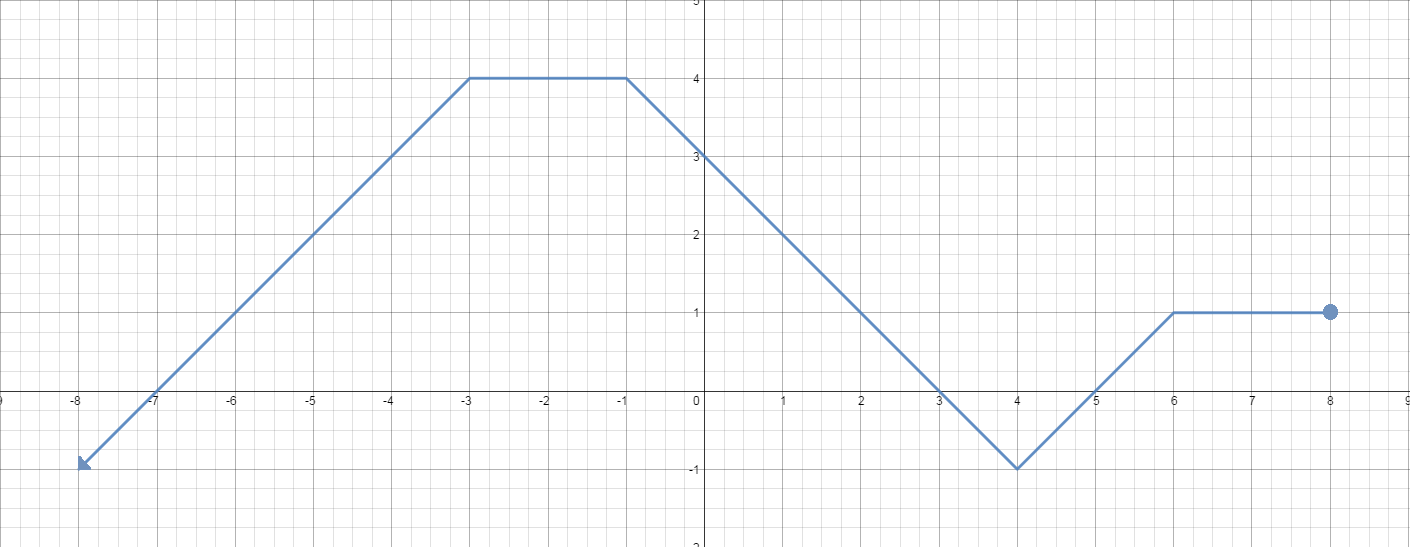# Square root of a function

### Square root of a function

What will happen if we put a square root in a function? What will its graph look like? We will tackle these questions in this lesson and see it yourself!

#### Lessons

• 1.
Comparing: $y = f\left( x \right)$ VS. $y = \sqrt {f\left( x \right)}$
Given $f\left( x \right) = x + 3$,
a)
Use a table of values, graph the functions $y = f\left( x \right)$ and $y = \sqrt {f\left( x \right)}$ .  $x$ $y = f\left( x \right) = x + 3$ $y = \sqrt {f\left( x \right)} = \sqrt {x + 3}$ -5 -4 -3 -2 1 6

b)
State the domain and range of each function.

• 2.
Graphing $y=\sqrt{f(x)}$ from the Graph of $y=f(x)$
Given the graph of $y = f\left( x \right)$ as shown:a)
Sketch the graph of $y = \sqrt {f\left( x \right)}$ .

b)
State the domain and range of:
i) $y = f\left( x \right)$
ii) $y = \sqrt {f\left( x \right)}$